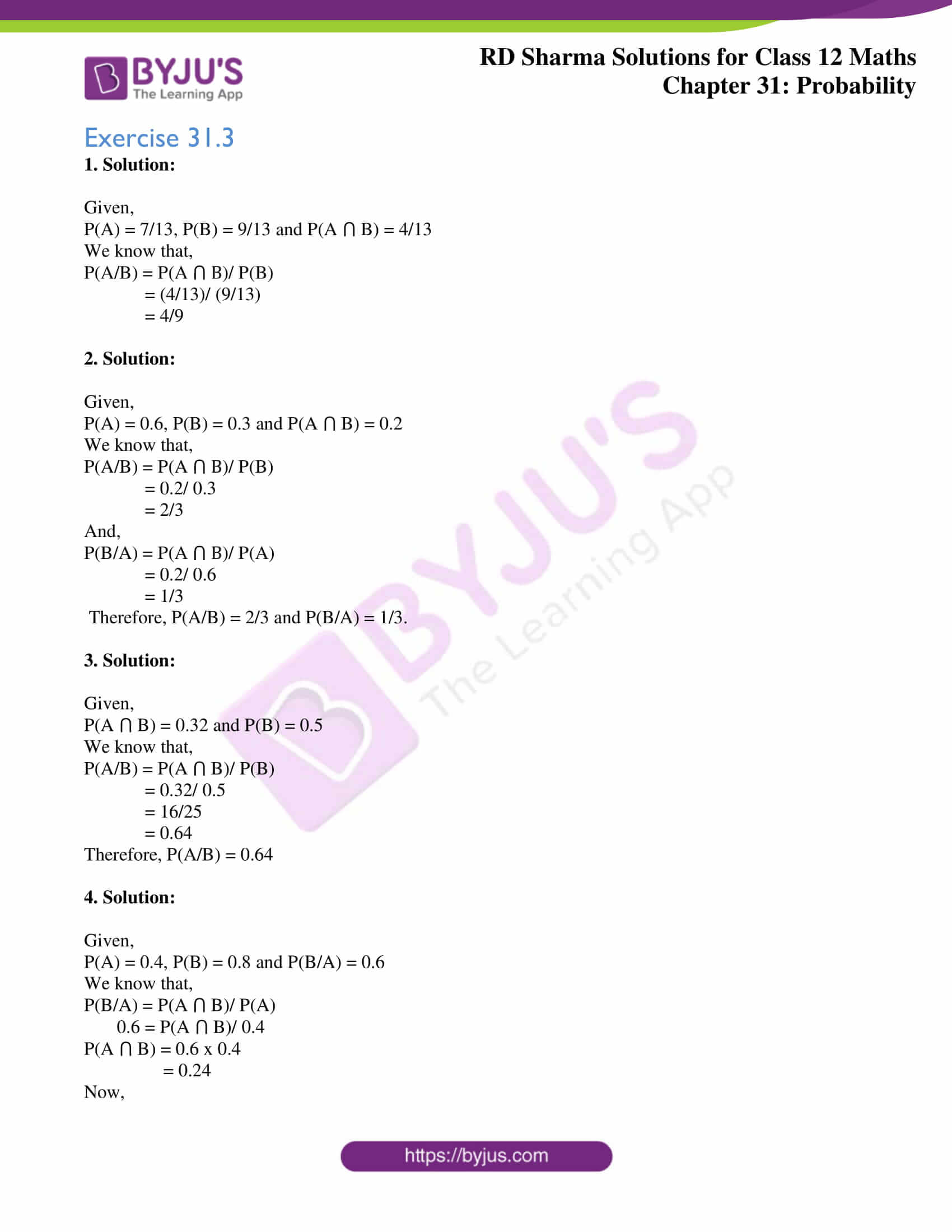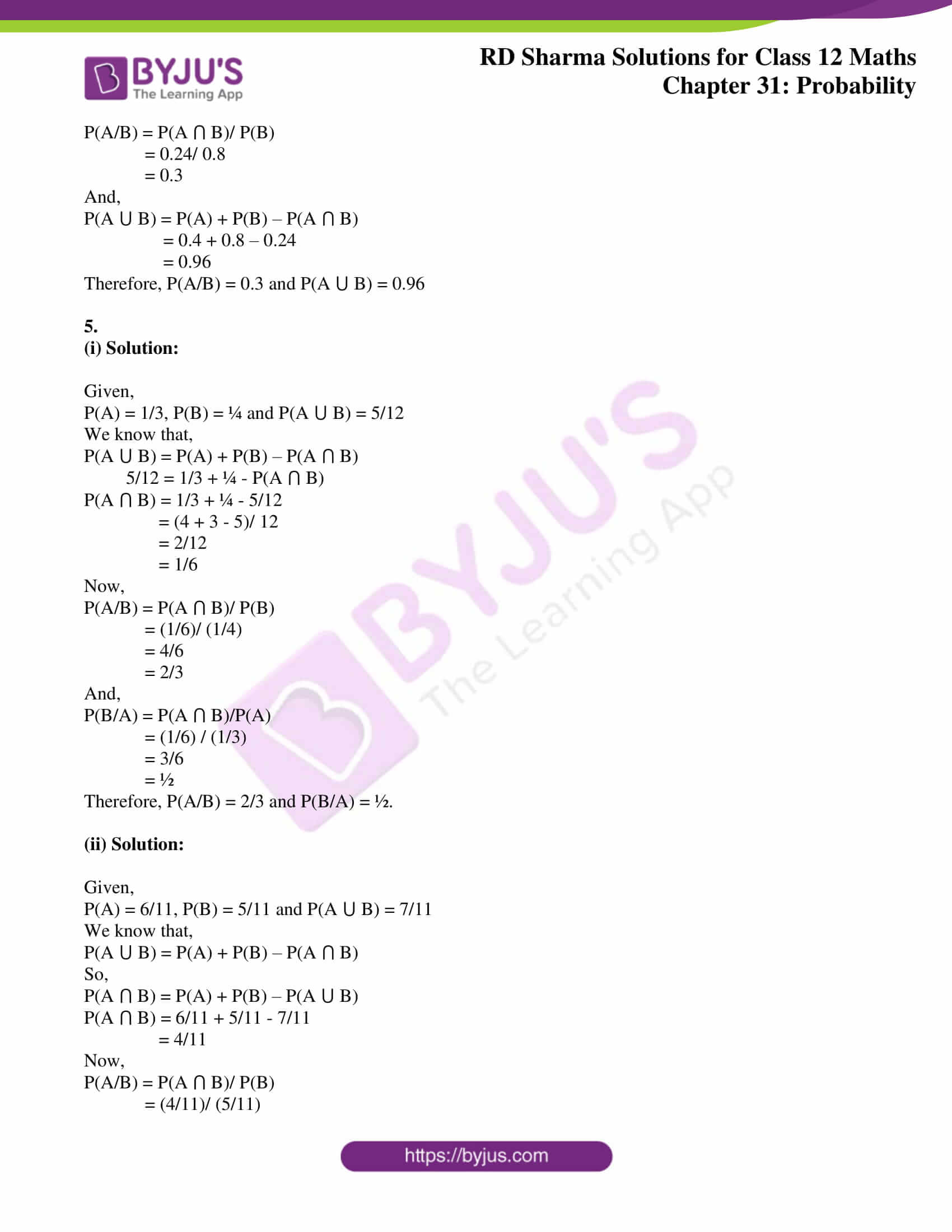# RD Sharma Solutions Class 12 Probability Exercise 31.3

RD Sharma Solutions for Class 12 Maths Chapter 31 Probability Exercise 31.3 can be accessed here. These solutions help students to grasp the concepts quickly and also improve their problem-solving skills. Individual subject matter experts have framed and solved the exercise-wise problems accurately. The RD Sharma Solutions are completely in accordance with the latest CBSE marking schemes in order to help students score high marks in their board examination. Students can easily download the PDF of these exercise solutions, which are available in the link given below.

## Download PDF of RD Sharma Solutions for Class 12 Maths Chapter 31 Exercise 3### Access RD Sharma Solutions for Class 12 Maths Chapter 31 Exercise 3

Exercise 31.3

1. Solution:

Given,

P(A) = 7/13, P(B) = 9/13 and P(A ⋂ B) = 4/13

We know that,

P(A/B) = P(A ⋂ B)/ P(B)

= (4/13)/ (9/13)

= 4/9

2. Solution:

Given,

P(A) = 0.6, P(B) = 0.3 and P(A ⋂ B) = 0.2

We know that,

P(A/B) = P(A ⋂ B)/ P(B)

= 0.2/ 0.3

= 2/3

And,

P(B/A) = P(A ⋂ B)/ P(A)

= 0.2/ 0.6

= 1/3

Therefore, P(A/B) = 2/3 and P(B/A) = 1/3.

3. Solution:

Given,

P(A ⋂ B) = 0.32 and P(B) = 0.5

We know that,

P(A/B) = P(A ⋂ B)/ P(B)

= 0.32/ 0.5

= 16/25

= 0.64

Therefore, P(A/B) = 0.64

4. Solution:

Given,

P(A) = 0.4, P(B) = 0.8 and P(B/A) = 0.6

We know that,

P(B/A) = P(A ⋂ B)/ P(A)

0.6 = P(A ⋂ B)/ 0.4

P(A ⋂ B) = 0.6 x 0.4

= 0.24

Now,

P(A/B) = P(A ⋂ B)/ P(B)

= 0.24/ 0.8

= 0.3

And,

P(A ⋃ B) = P(A) + P(B) – P(A ⋂ B)

= 0.4 + 0.8 – 0.24

= 0.96

Therefore, P(A/B) = 0.3 and P(A ⋃ B) = 0.96

5.

(i) Solution:

Given,

P(A) = 1/3, P(B) = ¼ and P(A ⋃ B) = 5/12

We know that,

P(A ⋃ B) = P(A) + P(B) – P(A ⋂ B)

5/12 = 1/3 + ¼ – P(A ⋂ B)

P(A ⋂ B) = 1/3 + ¼ – 5/12

= (4 + 3 – 5)/ 12

= 2/12

= 1/6

Now,

P(A/B) = P(A ⋂ B)/ P(B)

= (1/6)/ (1/4)

= 4/6

= 2/3

And,

P(B/A) = P(A ⋂ B)/P(A)

= (1/6) / (1/3)

= 3/6

= ½

Therefore, P(A/B) = 2/3 and P(B/A) = ½.

(ii) Solution:

Given,

P(A) = 6/11, P(B) = 5/11 and P(A ⋃ B) = 7/11

We know that,

P(A ⋃ B) = P(A) + P(B) – P(A ⋂ B)

So,

P(A ⋂ B) = P(A) + P(B) – P(A ⋃ B)

P(A ⋂ B) = 6/11 + 5/11 – 7/11

= 4/11

Now,

P(A/B) = P(A ⋂ B)/ P(B)

= (4/11)/ (5/11)

= 4/5

And,

P(B/A) = P(A ⋂ B)/P(A)

= (4/11) / (6/11)

= 4/6

= 2/3

Therefore, P(A/B) = 4/5 and P(B/A) = 2/3.